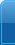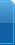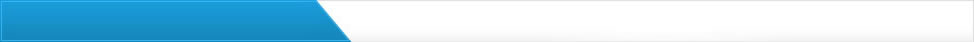浅析深基坑支护工程设计

1、工程简介
某工程位于市区的的主要道路，管段总长约28公里。根据该市环保局的设计要求并多方

2、工程地质情况
该深基路段位于河岸边,地表为杂填土，下卧冲洪积层,基底为砂岩。地下水与东平河有

3、支护结构设计
3.1 支护结构方案的选择
根据周围环境及地质条件，设计中拟定了钢板桩、灌注桩两种支护结构方案，并作了可

3.2 支护结构设计参数的选取
因作临时支护用，深基坑采用桩径d=80cm钻孔灌注桩，桩距为L=1.4米，桩身采用C20砼
，带肋钢筋。设计参数取：r=18KN/m3,θ=30o,地基基础承载力[R]=200KPa,基坑内侧地面均

3.3 设计实例
3.3.1 计算土压力
(1) 主动土压力
pa=yhk=18×8×tg2(45°－30°)=48.00KN
(2)总土压力
Ea=1/2•PaHL(1＋2h/H) (h 为荷载换算成成土柱高度，计算得h=0.633)
Ea=1/2×48×8×1.4×(1＋2×0.633/8)=313.354KN
作用点: ZEU=(H2＋3hH)/3×(2h＋H)=(82＋3×0.63×8)/3×(2×0.63＋8)=2.848米
3.3.2 建筑物荷载引起的侧压力
PS=KWSLS=0.333×25×9.8×2=163.17KN
K=tg2(45°－30°/2)=0.333
取LS=2米作用点:WPS=20/1.2=16.66米(<20米不作考虑)

3.3.3 求桩的锚固深度H1
根据土压力简图， 以C点为作用点，列平衡方程
∑xc=0
即: Ea－1/2×dh1σ=0 (d为桩径)
取σ=4[R]=4×200=800KN/m2
h1=2Ea/dσ=2×313.354/0.8×4×200=0.979米
(2) 求h2
由平衡方程∑MD=0得
Ea(ZEA＋h1＋h2)－1/2×dh1σ×(h2＋h1/3×)－2/3×d×σ×h2 2=0
即：
313.354×(2.848＋0.979＋h2)－1/2×0.8×0.979×800×(h2＋0.979/3)－2/3×0.8×800
×h2 2=0
整理得：426.667h2 2＋0.074h2－1096.972=0
426.667h2 2＋0.074h2 －1096.972=0
解方程得：h2=1.603m
H1=h1＋2h2=0.979＋2×1.603=4.198米

3.3.4 桩身配筋计算
(1)求最大弯矩点Mc
MC=313.354×(2.848＋0.979)－1/2×800×0.979×0.979/3=1071.414KN.m
(2)配筋计算
按等效刚度法，视d=0.8m的圆截面为b=h=0.9d的矩形截面梁
即b=h=0.9×0.8=0.72m
ho=0.92－0.04=0.86m
截面抵抗矩系数：Ao=rcMc/Rabho=(1.25×1071.41×106)/(11×720×680)=0.366
yo=0.5(1＋√1－2Ao)=0.5×(1＋√1－2×0.366)=0.898
AS=YSMC/YoRgho=1.25×1071.414×10/0.898×340×680=6450.645mm2
按不对称方法配筋，实际配受力钢筋：
10Φ25Ⅱ级钢筋 As1=4910mm2。配非受力钢筋： 10Φ14Ⅱ级钢筋As2=1540mm2，As实
=As1＋ As2，As实=6450mm2。
桩的配筋见下图：
4、应急措施
(1) 在地面以下约2.5m的深度范围内采取自然放坡，只作空心护壁不作桩心，减少桩的

(2) 为确保万无一失，施工中可进行局部预挖试验。(3) 基底标高以上30厘米厚度的土

(4) 堵住流向基坑的地面及地下水，在发现泄水的地方，一般可采用常规的水导管、抽

(5) 严格按有关施工规范和验收规程操作。
5、结语
挡土桩与其它深基础支护工程相比，具有安全、可靠，造价适中、周期短、施工方便等

参考文献：
 JGJ120－99，建筑基坑支护技术规范[S].
 《土力学地基与基础》
 《基础工程》
 《建筑地基与基础设计规范》（GB 50007－2002）[S] 中国建筑工业出版社 2002.4